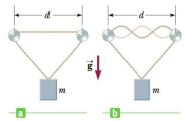Chapter 14, Problem 49P

Chapter
Section
Textbook Problem

A 12.0-kg object hangs in equilibrium from a string with total length of L = 5.00 m and linear mass density of μ = 0.001 00 kg/m. The string is wrapped around two light, frictionless pulleys that are separated by the distance d = 2.00 m (Fig. P14.49a). (a) Determine the tension in the string. (b) At what frequency must the string between the pulleys vibrate in order to form the standing-wave pat tern shown in Figure P14.49b?Figure P14.49

(a)

To determine
The tension in the string.

Explanation

Given Info: the total length of the string is L=5.00m and separated distance between the pulleys is d=2.00m .

From the given figure, the change in length of the string is,

L=Ld2

• L is the change in length,
• L is the total length of the string,
• d is the separated distance of the pulleys,

Substitute 5.00m for L and 2.00m for d .

L=5.00m2.00m2=1.50m

From the given figure, the angle is,

θ=sin1(d/2L)

• θ is the angle between separated distance and change in length of the string.

Substitute 1.50 m for L and 2.00m for d .

θ=sin1(2.00m/21.50m)=41

(b)

To determine
The frequency of the string between the pulleys vibrate in the standing wave pattern shown in the given figure.

Still sussing out bartleby?

Check out a sample textbook solution.

See a sample solution

The Solution to Your Study Problems

Bartleby provides explanations to thousands of textbook problems written by our experts, many with advanced degrees!

Get Started